# Cross Product

Three children are pulling a rope in different directions.

Each child uses a certain amount of force to pull the rope.

What will be the force exerted by each child?

Can we determine them?

Consider two arrows $$a$$ and $$b$$.

The cross product of these two arrows is defined as the third arrow that is perpendicular to the two original arrows.

Its magnitude is given by the area of the parallelogram between them and its direction can be determined by the thumb rule.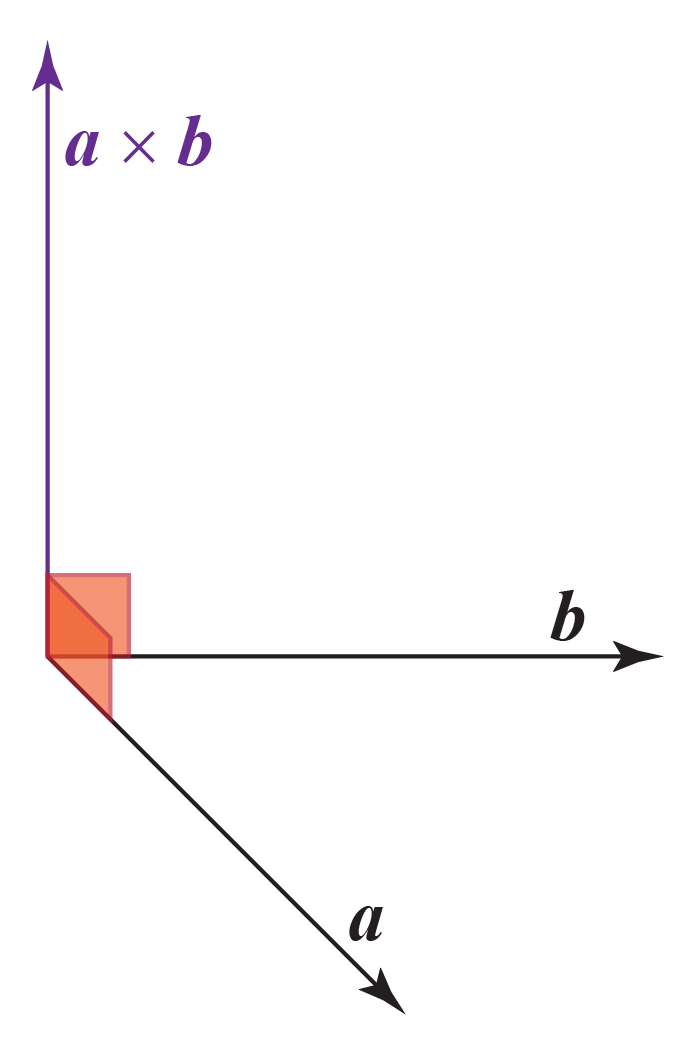In this mini-lesson on cross product, we can learn about cross product meaning, cross product example, cross product formula, and cross product rules.

## Lesson Plan

 1 What Is a Cross Product? 2 Important Notes on Cross Product 3 Tips and Tricks on Cross Product 4 Solved Examples on Cross Product 5 Interactive Questions on Cross Product

## What Is a Cross Product?

A vector has both magnitude and direction.

We can multiply two or more vectors by cross product and dot product.

When two vectors are multiplied with each other and the product is also a vector quantity, then the resultant vector is called the cross product or the vector product.

The $$\times$$ symbol is used between the original vectors.

$$\overrightarrow{a} \times \overrightarrow{b} = \overrightarrow{c}$$

### Cross Product Example

Cross product plays a crucial role in several branches of science and engineering.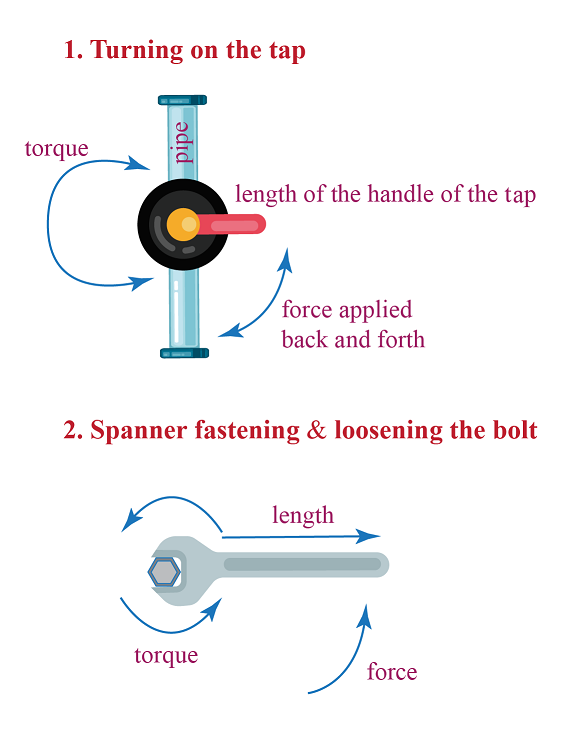Example 1: Turning on the tap:

We apply equal and opposite forces at the two diametrically opposite ends of the tap. Torque is applied in this case.

In vector form, torque is the cross product of the radius vector (from axis of rotation to the point of application of force) and the force vector.

i.e. $$\overrightarrow{T} = \overrightarrow{r} \times \overrightarrow{F}$$

Example 2: Twisting a bolt with a spanner:

The length of the spanner = one vector

The direction we apply force on the spanner (to fasten or loosen the bolt) = another vector

The resultant twist direction is perpendicular to both the vectors.

## What Is the Cross Product of Two Vectors?

Let us assume that $$\overrightarrow{a}$$ and $$\overrightarrow{b}$$ are two vectors, such that

$$\overrightarrow{a}$$= $$a_1i+a_2j+a_3k$$ and

$$\overrightarrow{b}$$ = $$b_1i+b_2j+b_3k$$

Using determinants, we could find the cross product and write the result as shown in the picture below.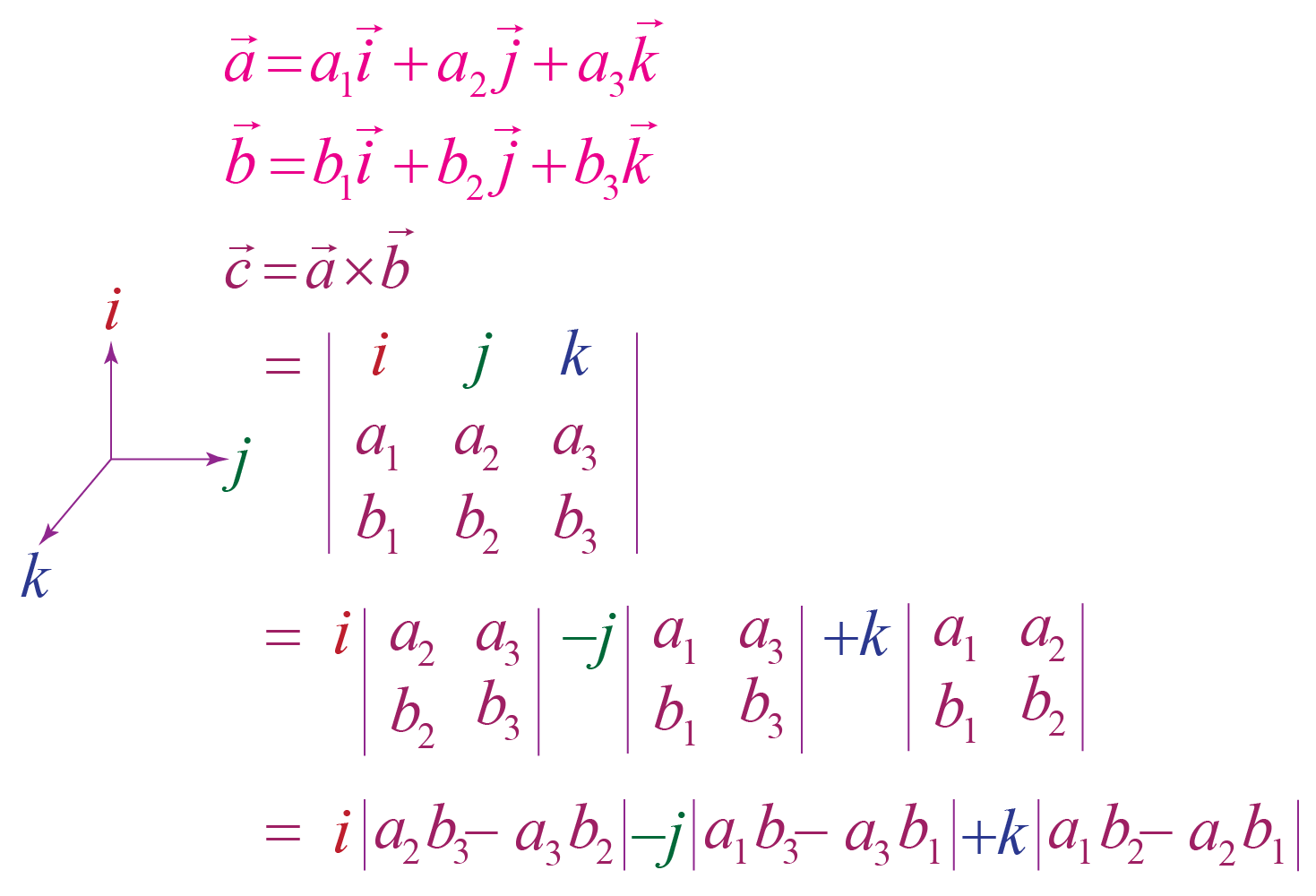### Cross Product Formula

The cross product of the two vectors is represented as

 $$\overrightarrow{a} \times \overrightarrow{b} = i(a_2b_3-a_3b_2) \\- j(a_1b_3-a_3b_1)\\+k(a_1b_2-a_2b_1)$$

### Cross Product Meaning

Use the simulation below and observe the angles between the vectors$$\overrightarrow{a}$$ and $$\overrightarrow{c}$$ and the angles between the vectors $$\overrightarrow{b}$$ and $$\overrightarrow{c}$$.

Also verify if you can position vectors $$\overrightarrow{a}$$ and $$\overrightarrow{b}$$ to get a zero vector.

Try to observe how you can position the original vectors to get the greatest magnitude.

• The angle between $$\overrightarrow{a}$$ and $$\overrightarrow{c}$$ is always 90$$^\circ$$.

i.e., $$\overrightarrow{a}$$ and $$\overrightarrow{c}$$ are orthogonal vectors.

The angle between $$\overrightarrow{b}$$ and $$\overrightarrow{c}$$ is always 90$$^\circ$$.

i.e., $$\overrightarrow{b}$$ and $$\overrightarrow{c}$$ are orthogonal vectors.

• We can position $$\overrightarrow{a}$$ and $$\overrightarrow{b}$$ in such a way that there is no angle between them, making the resultant vector a zero vector.
• To get the greatest magnitude, the original vectors must be perpendicular so that the cross product will be maximum.

## What Is The General Formula of Cross Product?

Consider 2 vectors.

$$\overrightarrow{a} = a_1i+a_2j+a_3k$$ and

$$\overrightarrow{b} = b_1i+b_2j+b_3k$$

Let $$\theta$$ be the angle formed between $$\overrightarrow{a}$$ and $$\overrightarrow{b}$$ and $$n$$ is the unit vector perpendicular to both $$\overrightarrow{a}$$ and $$\overrightarrow{b}$$ to head their directions correctly.

The magnitude of the vectors is given by the area of the parallelogram that is spanned by the two vectors.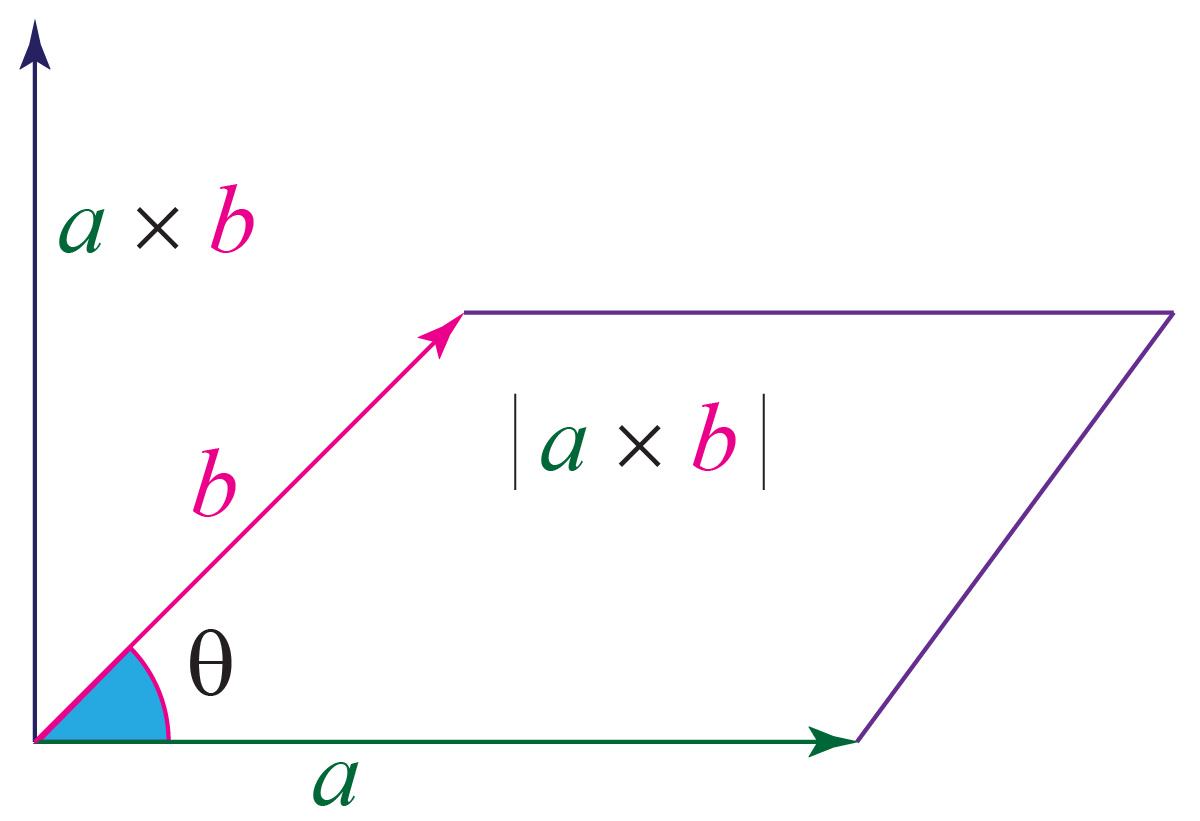The length of the cross product of the two vectors is given by the formula:

 $$\overrightarrow{a} \times \overrightarrow{b} = |a| |b| \sin(\theta) n$$

Where$$\mid a \mid$$ is the magnitude or the length of $$\overrightarrow{a}$$

$$\mid b \mid$$ is the magnitude or the length of $$\overrightarrow{b}$$

If you point your right hand in the direction of the first arrow and curl your fingers in the direction of the second, then your thumb will end up pointing in the direction of the cross product.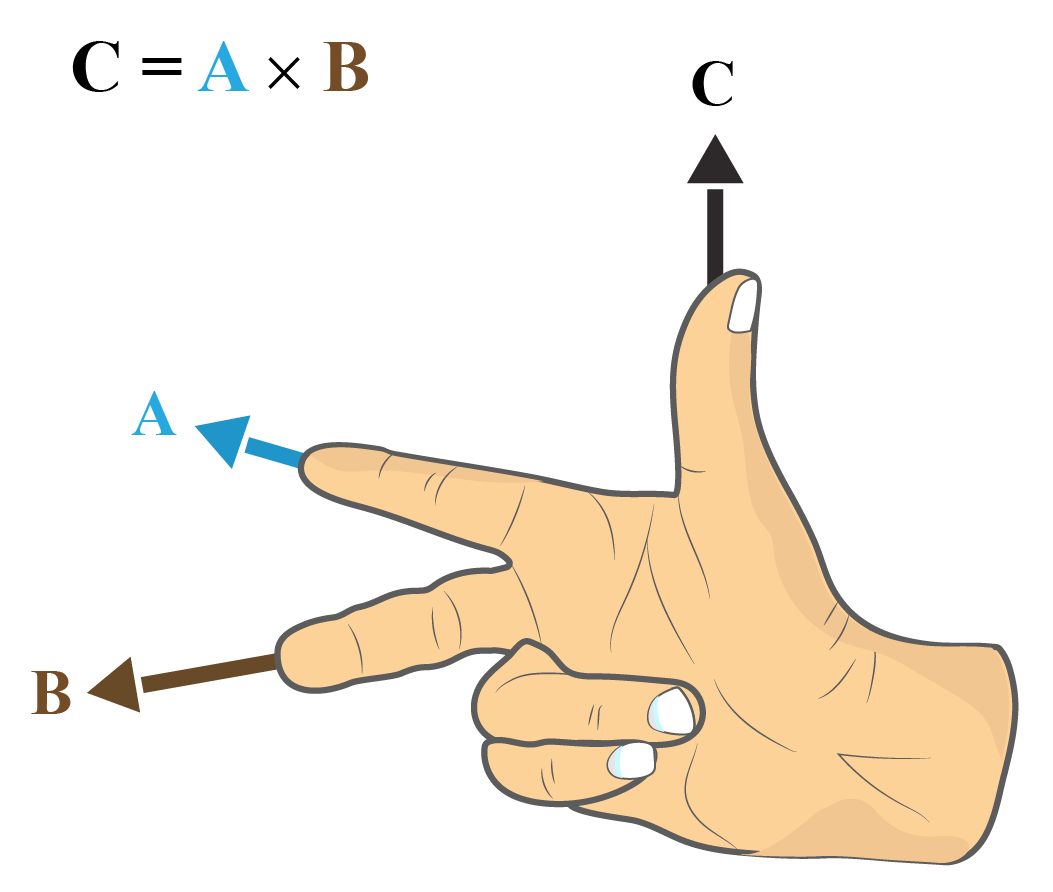### Cross Product Examples

Calculate the area of the parallelogram formed by the vectors a=(3,−3,1) and b=(4,9,2)

The cross product is given as

\begin{align}a \times b &= \begin{matrix} i & j & k\\ 3 & -3 & 1\\ 4 & 9 & 2 \end{matrix}\\\\&=[(-3\times2) - (1\times9)]\\ -&[(3\times 2) - (1\times 4)]\\ +&[(3\times 9) -(-3\times 4)]\\\\&=(-6-9) - (6-4) + (27+12)\\&=-15\overrightarrow{i}-2\overrightarrow{j}+39\overrightarrow{k}\end{align}

\begin{align}\text{Area of the parallelogram }&= \sqrt{15^2+2^2+39^2}\\\\&=\sqrt{1750}\\\\&= 5\sqrt{70}\text{sq units.}\end{align}

## What Are the Rules of Cross Product?

The cross product rules are as follows:

1. The length of the cross product of the two vectors $$= \overrightarrow{a} \times \overrightarrow{b} = |a| |b| \sin(\theta)$$.
2. Anti-commutative property: $$\overrightarrow{a} \times \overrightarrow{b} = - \overrightarrow{b} \times \overrightarrow{a}$$
3. Distributive property: $$\overrightarrow{a} \times (\overrightarrow{b} + \overrightarrow{c})\\ = (\overrightarrow{a}\times \overrightarrow{b} )+ (\overrightarrow{a}\times \overrightarrow{c})$$
4. Cross product of the zero vector: $$\overrightarrow{a}\times \overrightarrow{0} = \overrightarrow{0}$$
5. Cross product of the vector with itself: $$\overrightarrow{a}\times \overrightarrow{a} = \overrightarrow{0}$$
6. Multiplied by a scalar quantity:$$\overrightarrow{c}(\overrightarrow{a}\times \overrightarrow{b}) = c\overrightarrow{a}\times \overrightarrow{b} = \overrightarrow{a}\times c\overrightarrow{b}$$
7. The cross product of the unit vectors: $$\overrightarrow{i}\times \overrightarrow{i} =\overrightarrow{j}\times \overrightarrow{j} = \overrightarrow{k}\times \overrightarrow{k} = 0$$
8. $$\overrightarrow{i}\times \overrightarrow{j} = \overrightarrow{k}\\ \overrightarrow{j}\times \overrightarrow{k}= \overrightarrow{i}\\\overrightarrow{k}\times \overrightarrow{i} = \overrightarrow{j}$$
9. $$\overrightarrow{j}\times \overrightarrow{i} = \overrightarrow{-k}\\ \overrightarrow{k}\times \overrightarrow{j}= \overrightarrow{-i}\\ \overrightarrow{i}\times \overrightarrow{k} = \overrightarrow{-j}$$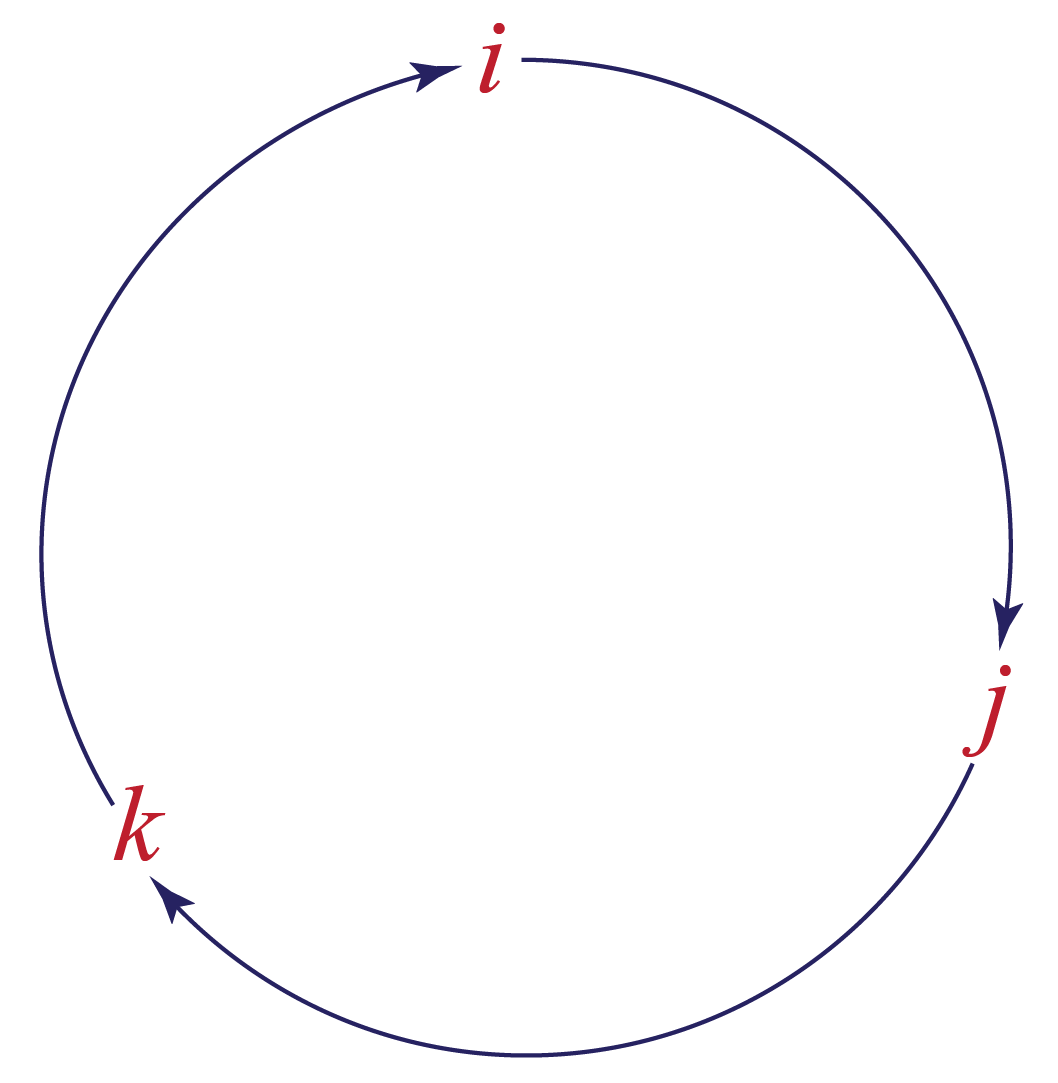More Important Topics
Numbers
Algebra
Geometry
Measurement
Money
Data
Trigonometry
Calculus